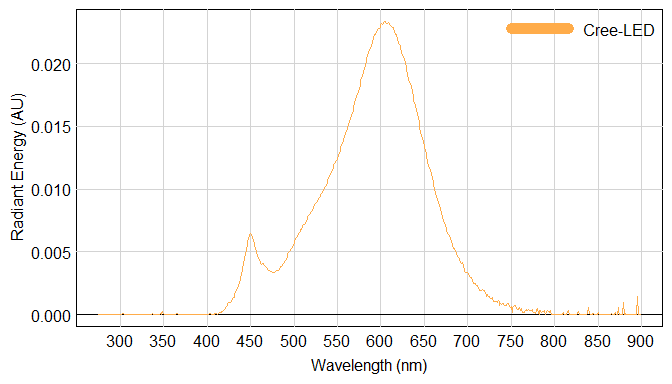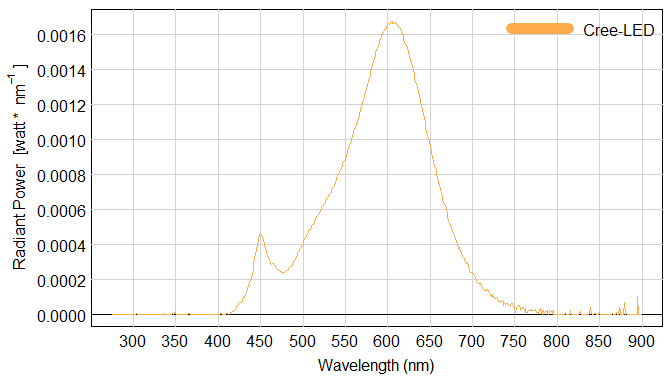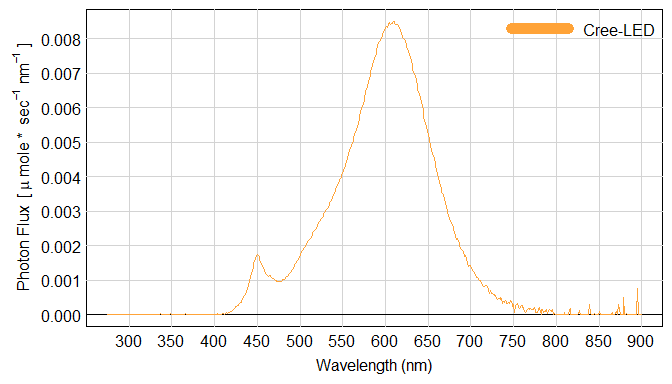# Photon Counting

#### 2022-05-03

This colorSpec vignette is a response to the question:

Is there any program that given the lumen and the colour spectrum (relative intensity at any wavelength) of a light source and it outputs the total amount of photons per wavelength?

that was asked on a stackexchange forum, see . Featured functions in this vignette are: actinometric() and photometric().

library( colorSpec )

Read the SPD for a domestic LED bulb from the Lamp Spectral Power Distribution Database, see . The unique ID is LED_A19_dim_domestic-use_softwhite-color_CREE-brand_6W_120V_75Lum_2700K_bernard.

wave = 275:899
path = system.file( "extdata/sources/Cree-LED.txt", package="colorSpec" )
bulb = readSpectra( path, wavelength=wave )
par( omi=c(0,0,0,0), mai=c(0.6,0.8,0.1,0.1) )
plot( bulb, main='' )Figure 1. Energy Spectrum of a Domestic LED Bulb, manufactured by Cree

The energy unit is unknown. But it does not matter, because we are now going to scale so that the luminous flux of the SPD is 75 lumens, as specified by the manufacturer.

luminous.flux = photometric( bulb ) ; luminous.flux
##                 photopic1924 scotopic1951 photopic1978 photopic2008
## Cree-LED.energy     1046.456     1193.872     1047.376     1101.321

The unit is lumen, but the first one - photopic1924 - is the appropriate official standard. Scale spectrum to have 75 lumens.

bulb = multiply( bulb, 75/luminous.flux ) ; bulb
##
## colorSpec object.   The organization is 'df.col'.  Object size is 13120 bytes.
## the object describes a single source of light, and the quantity is 'energy' (energy of photons, which is radiometric).
## Wavelength range: 275 to 899 nm.  Step size is 1 nm.
##
## 1 spectra
## 625 data points / spectrum
##
##            Source Min         Max LambdaMax  Integral
## 1 Cree-LED.energy   0 0.001675584       605 0.2235534
#   check that the luminous flux is now 75 lumens
photometric( bulb )
##                 photopic1924 scotopic1951 photopic1978 photopic2008
## Cree-LED.energy           75     85.56539     75.06593      78.9322

From the man page for photometric() we know that the unit for bulb is $$\textrm{watt} * \textrm{nm}^{-1}$$.

par( omi=c(0,0,0,0), mai=c(0.6,0.95,0.1,0.1) )
ylab = expression( 'Radiant Power'  ~~~  '[watt * ' ~ nm^-1 ~ ']' )
plot( bulb, main='', ylab=ylab )Figure 2. Energy Spectrum of a Domestic LED Bulb, manufactured by Cree

## Convert from an Energy-Based to a Photon-Based Spectrum

The unit is currently energy-based (energy of photons, aka radiometric), but we need photon-based (number of photons, aka actinometric). From the man page for actinometric() we know that the output unit is $$\mu \textrm{mole} * \textrm{sec}^{-1} * \textrm{nm}^{-1}$$.

bulb = actinometric( bulb )
par( omi=c(0,0,0,0), mai=c(0.6,0.9,0.1,0.1) )
ylab = expression( 'Photon Flux'  ~~~  '[' ~ mu ~ 'mole * ' ~ sec^-1 ~ nm^-1 ~ ']'  )
plot( bulb, main='', ylab=ylab )Figure 3. Photon Flux of a Domestic LED Bulb, manufactured by Cree

This plot looks similar but note that $$\lambda_{max}$$ has moved slightly higher. For the total number of photons/sec from this bulb, compute the integral over $$\lambda$$.

bulb
##
## colorSpec object.   The organization is 'df.col'.  Object size is 13120 bytes.
## the object describes a single source of light, and the quantity is 'photons' (number of photons, which is actinometric).
## Wavelength range: 275 to 899 nm.  Step size is 1 nm.
##
## 1 spectra
## 625 data points / spectrum
##
##            Source Min         Max LambdaMax Integral
## 1 Cree-LED.energy   0 0.008500948       609 1.102559

So the total photon flux of the bulb is $$1.102559 ~ \mu \textrm{mole/sec}$$. To convert this to exaphotons, multiply by $$0.602214 ~ \textrm{exaphotons/} (\mu \textrm{mole of photons} )$$ to get $$0.663976 ~ \textrm{exaphotons/sec}$$.

## Session Information

R version 4.2.0 (2022-04-22 ucrt)
Platform: x86_64-w64-mingw32/x64 (64-bit)
Running under: Windows 10 x64 (build 19044)

Matrix products: default

locale:
 LC_COLLATE=C
 LC_CTYPE=English_United States.utf8
 LC_MONETARY=English_United States.utf8
 LC_NUMERIC=C
 LC_TIME=English_United States.utf8

attached base packages:
 stats     graphics  grDevices utils     datasets  methods   base

other attached packages:
 spacesRGB_1.4-0 colorSpec_1.4-0

loaded via a namespace (and not attached):
 digest_0.6.29        R6_2.5.1             jsonlite_1.8.0
 magrittr_2.0.3       evaluate_0.15        highr_0.9
 rlang_1.0.2          stringi_1.7.6        jquerylib_0.1.4
 bslib_0.3.1          rmarkdown_2.14       tools_4.2.0
 stringr_1.4.0        xfun_0.30            yaml_2.3.5
 fastmap_1.1.0        compiler_4.2.0       microbenchmark_1.4.9
 htmltools_0.5.2      knitr_1.39           sass_0.4.1